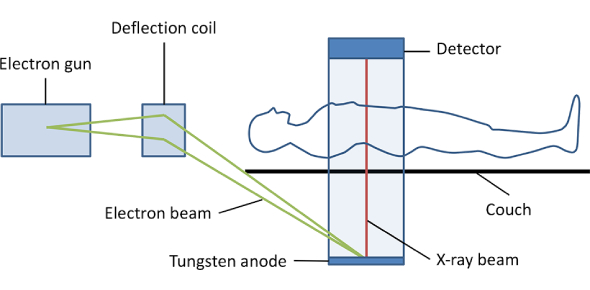# CT Physics And Instrumentation

36 Questions | Total Attempts: 7556SettingsA Computerized Tomography is an x-ray imaging procedure in which a narrow beam of x-rays is aimed at a patient and quickly rotated around the body. CT scanner uses a motorized x-ray source that rotates around the circular opening of a donut-shaped structure called a gantry. The quiz below is designed to help you revise what you know about CT physics and instrumentation.

• 1.
A pixel may be defined as
• A.

The portion of the CRT displaying the image

• B.

A volume element

• C.

A picture element

• D.

A miniature image

• 2.
The mathematical technique that involves the estimation of an unknown value from values on either side of it s known as
• A.

Filtering

• B.

Interpolation

• C.

Convolution

• D.

Summation

• 3.
Pixels representing tissues with average attenuation coefficients greater than that of water have which of the types of values?
• A.

Extremely small

• B.

Positive

• C.

Negative

• D.

None of the above

• 4.
When compared with conventional X-ray, CT produces diagnostic images with improved
• A.

Low contrast resolution

• B.

Spatial resolution

• C.

Minute detail

• D.

PT dose

• 5.
IN 1917, the Austrian mathematician _____________ proved that it was possible to reconstruct a three dimensional object from the infinite set of all progections.
• A.

• B.

Tsien

• C.

Bracewell

• D.

Cormack

• 6.
Which of the following is not commonly used as a CT scintillation detector
• A.

Ceramic rare earth

• B.

Silver halide

• C.

Bisumth germinate

• D.

• 7.
If a ROI is placed on an object of interest and the HU measures +1.9 HU, the material is most likely to be
• A.

Fat

• B.

Blood

• C.

Tumor

• D.

Water

• 8.
The average window width range for a abdomen is
• A.

200-250 HU

• B.

350-600 HU

• C.

650-850 HU

• D.

900-1200 HU

• 9.
What is the average linear attenuation coefficient coefficient for water?
• A.

.0007

• B.

.155

• C.

.206

• D.

.530

• 10.
Which of the following it the primary interaction between x-ray photons and tissue during CT examination
• A.

Bremsstrahlung effect

• B.

Characteristic effect

• C.

Compton effect

• D.

Coherent scatter

• 11.
Area of the CT image that contain minimal changes in tissue density are electronically represented by
• A.

Positive CT numbers

• B.

High spatial frequencies

• C.

Negative CT numbers

• D.

Low spatial frequencies

• 12.
Ring artifacts on the CT image are associated with which of the following tube detector relationship?
• A.

Rotate-nutate

• B.

Rotate-stationary

• C.

Rotate-rotate

• D.

Translate-rotate

• 13.
In the binary number system, a byte series of ___________ bits of information
• A.

2

• B.

4

• C.

8

• D.

16

• 14.
An accurate, modern CT scanner is capable of spatial resolution of up to
• A.

10 lp/mm

• B.

20 lp/mm

• C.

10 lp/cm

• D.

20 lp/cm

• 15.
Which of the following is the best method to reduce respiratory motion of the CT image?
• A.

Good PT-Tech communication

• B.

Reduced scan times

• C.

Use of immobilization devices

• D.

• 16.
Which of the following statements is correct regarding the radiographic film used to archive CT images1 It is double emulsion film2 It is sensitive to ultraviolet light only3 It may be used in conjunction with multiformat or laser camera
• A.

1 only

• B.

2 only

• C.

3 only

• D.

1 and 3

• 17.
Which of the following reconstruction methods is used by most modern CT scanners
• A.

Back projection

• B.

Iterative method

• C.

Fourier transform

• D.

Filtered back projection

• 18.
4th generation CT scanners use a ___________ tube-detector configuration
• A.

Rotate-translate

• B.

Electron beam-stationary

• C.

Rotate-stationary

• D.

Rotate-rotate

• 19.
The process by which electrons are produced at the cathode of a CT x-ray tube is know as
• A.

Rectification

• B.

Anode heel effect

• C.

Thermionic emission

• D.

Isotrophic emission

• 20.
Which of the following increases the signal-to-noise ratio of a CT image
• A.

Decreased aperture size

• B.

Decreased milliampere seconds MAS

• C.

Increased aperture size

• D.

Increased filtration

• 21.
A straight line appearing vertically on the scanogram of a fourth generation CT scanner is a artifact most likely caused by
• A.

• B.

Detector malfunction

• C.

Tube arching

• D.

Increased aperture size

• 22.
The acronym CTDI is used to describe which of the following
• A.

A specialized CT imaging technique used to measure bone mineral density

• B.

A quality control test that measures the accuracy of the laser lighting system

• C.

The radiation dose to the PT during the CT scan

• D.

A high speed CT scanner used for cardiac imaging

• 23.
What is the DFOV used for a 320x320 matrix image with a pixel dimension of .75 x .75 mm?
• A.

12cm

• B.

24cm

• C.

36cm

• D.

48cm

• 24.
The full width at half maximum of a CT scanner is used to describe
• A.

Spatial resolution

• B.

Contrast resolution

• C.

Noise

• D.

Calibration accuracy

• 25.
The average photon energy of the primary beam of a CT scanner operating at a tube potential of 120kvp is
• A.

50 keV

• B.

70 keV

• C.

100 keV

• D.

120 keV

Related TopicsBack to top